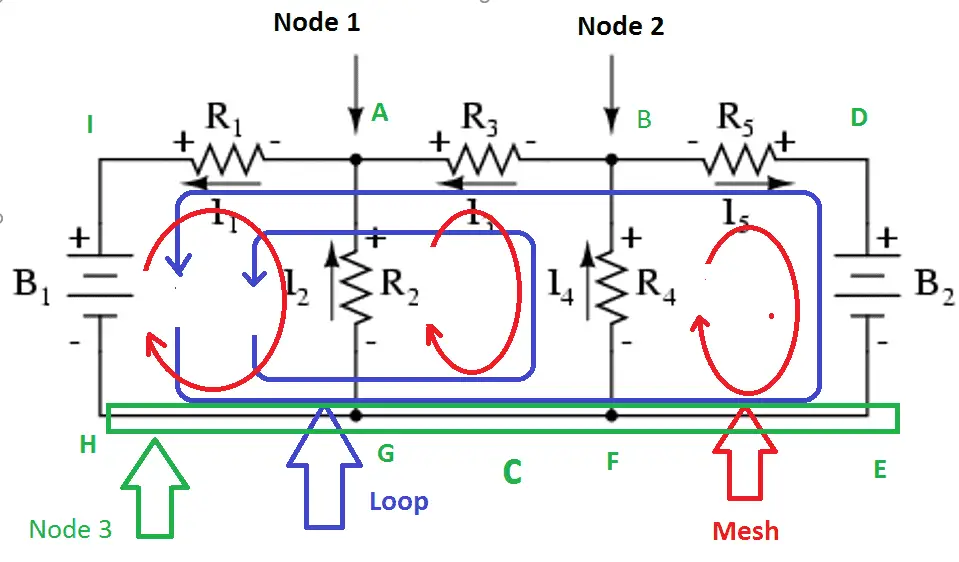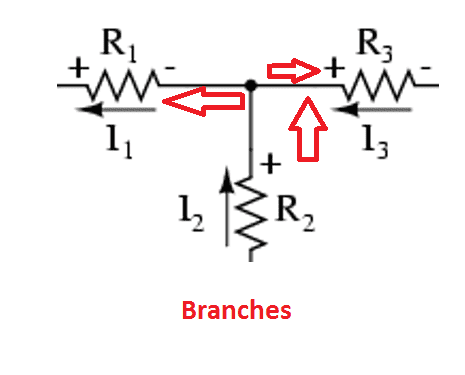# What is Electrical Network

## What is electrical network:

Network is the most used word in an electric or electronic circuit while solving a circuit problem or creating a balanced circuit. A network does not require to have a closed path, the open circuit also can be called as a network. In such a way all the networks are not a circuit (example: open circuit cannot be called as circuit and it can be called as a network) and all the circuit can be called as network. An electrical network can be identified by four circuit names such as Node, Branch, Loop and Mesh. Let see the detailed explanation of each one..

### Node

A junction point of two or more active components (voltage source, current sources) or passive components connection (resistor, inductor, capacitor etc) is called Node or in a network is an equipotential part at which two or more circuit’s element are joined.

### Branch

Branch is nothing but a part of an electric circuit which locates between two junctions is called branch. In branch, one or more elements can be connected and they have two terminals.

### Loop

Loop is nothing but A closed path of an electric circuit where one or more than two meshes can be followed i.e. there may be many meshes in a loop. To be a loop the circuit should be in the closed path. To be a complete circuit, the circuit must have at least one loop.

### Mesh

A mesh is a closed path that does not contain any other loops or A closed loop which contains no other loop within it or a path which does not contain on other paths is called Mesh.

## The best example for an Electrical network:

Let us consider below-mentioned diagram:### Nodes of the circuit:

In this, Point A and B can be called as nodes of the circuit, the circuit resistor R1,R2, and R3 are joined at a point A which is node 1; R3, R4, and R5 are joined in a point B which is node 2. And the battery 2 negative point, R4, R2 and Battery 1 negative terminal are joined at point C which is node 3.

Learn More:   Motor Hp (Horse Power) Calculator DC, Single Phase & Three phase

### Branches of the circuit:### Mesh of the circuit:

Mesh is nothing but a single closed path, in given circuit, we have three meshes.

Mesh1: IAGHI,

Mesh2: ABFGA

Mesh3: BDEFB

### Loop of the circuit:

Loop is nothing but a closed path of the circuit. The given circuit we can find 5 different loops,

Loop1: IAGHI,

Loop2: ABFGA

Loop3: BDEFB

Loop4: IABFGHI

Loop5: IABDEFGHI

1.Abubakar saleh tataru
2.oscar james